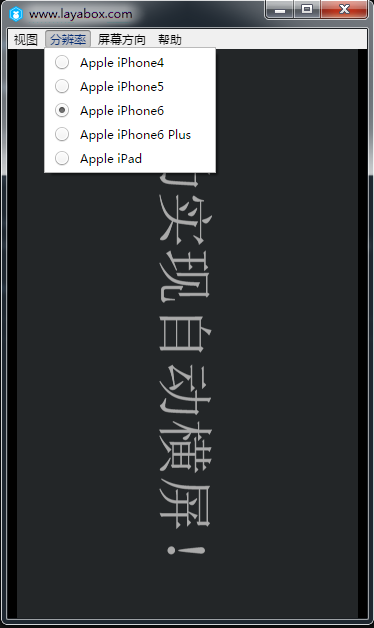# 自动横屏与横屏游戏（JS）

711501594

LayaAir引擎如何设置自动横屏

 12345678910111213141516171819202122232425262728293031323334 `(``function``()``{``    ``var` `Stage = Laya.Stage;``    ``var` `Text  = Laya.Text;`` ` `    ``(``function``()``    ``{``        ``//初始化舞台``        ``Laya.init(500, 300);``        ``//让舞台处于屏幕的垂直居中``        ``Laya.stage.alignV = Stage.ALIGN_MIDDLE;``        ``//让舞台处于屏幕的水平居中``        ``Laya.stage.alignH = Stage.ALIGN_CENTER;``        ``//保持原始高宽比的情况下，将舞台铺满屏幕，超出比例的部分会有黑边``        ``Laya.stage.scaleMode = Stage.SCALE_SHOWALL;``        ``//`自动横屏，舞台的水平方向与屏幕的最短边保持垂直。`        ``Laya.stage.screenMode = Stage.SCREEN_HORIZONTAL;``        ``//设置舞台背景色``        ``Laya.stage.bgColor = ``"#232628"``;``        ``showText();``    ``})();`` ` `    ``function` `showText()``    ``{``        ``var` `text = ``new` `Text();``        ``text.text = ``"成功实现自动横屏！"``;``        ``text.color = ``"gray"``;``        ``text.fontSize = 50;``        ``text.x = Laya.stage.width - text.width >> 1;``        ``text.y = Laya.stage.height - text.height >> 1;` `        ``Laya.stage.addChild(text);``     ``}``})();`